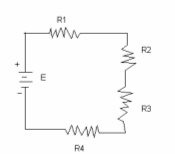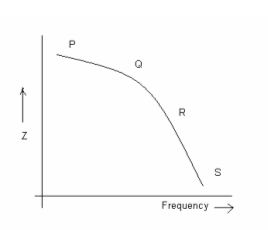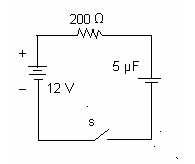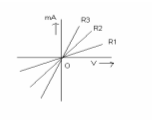# RLC Circuits - Electronics Engineering test questions

## RLC Circuits - Electronics Engineering test questions

(1) A capacitor can be used for

(A) Both a.c. and d.c.
(B) d.c. but blocks a.c.
(C) a.c. but blocks d.c.
(D) None of the above

(2) Consider an inductor that passes a current of 7.96 mA. What will be the value of inductor when it is supplied with 50 V ac with a frequency of 10 kHz?

(A) 100 mH
(B) 10 mH
(C) 1 mH
(D) 1H.

(3) Let a source of 220V, 5Hz is connected to an electric bulb whose rating is 220 V. Then, the bulb

(A) Glows intermittently
(B) Glows continuously
(C) Fuses
(D) Does not glow

(4) The secondary voltage is 1V when a 100 mA alternating current with a frequency of 1 kHz flows in the primary of two coupled coils. The mutual inductance between the coils is

(A) 3.18 mH
(B) 31.8 mH
(C) 15.9 mH
(D) 1.59 mH

(5) _____________ refers to the parallel circuit

(A) The voltage across element is in proportion to its resistance value
(B) The equivalent resistance is greater than any one of the resistors
(C) The current through any one element less than the source current
(D) The current through each element is same

ANSWER: The current through any one element less than the source current

(6) The capacitor will complete its discharge most rapidly in

(A) 10 μF capacitor with a 10 V charge, discharging through a 1 kΩ resistor
(B) A 50 pF capacitor with a 20 mV charge discharging through a 200 milli-ohm resistance
(C) 10 μF capacitor with a 50 V charge discharging through a 1 kΩ resistor
(D) Discharge rate will be same in all cases.

ANSWER: A 50 pF capacitor with a 20 mV charge discharging through a 200 milli-ohm resistance

(7) For the diagram shown below, under which of the following case the power dissipated in R3 will increase?(A) When R1 is increased
(B) When R2 is doubled
(C) E is reduced
(D) R4 is short circuited.

(8) Capacitive susceptance is a measure of

(A) The extent of neutralization of reactive power in a circuit
(B) A purely capacitive circuit's ability to resist the flow of current
(C) A purely capacitive circuit's ability to pass current
(D) Reactive power in a circuit.

ANSWER: A purely capacitive circuit's ability to pass current

For Questions 9 to 12 refer to data given below:

Resistance R = 20Ω, Inductance L = 20mH are connected in series with an ac supply of 60 V with f = 100Hz.

(9) The current in R is

(A) 2.54 A
(B) 5.08 A
(C) 1.27 A
(D) 10.26 A

(10) The voltage across R is

(A) 30.6 V
(B) 50.8 V
(C) 24.4 V
(D) 40.8 V

(11) The voltage across L is

(A) 50.5
(B) 45.5 V
(C) 39.1 V
(D) 31.9 V

(12) The phase angle of current with respect to supply voltage is

(A) 20.1°
(B) 40.1°
(C) 32.1°
(D) 28.1°

(13) From the options given below which one represents largest mmf?

(A) A 100-turn coil wound around a 30-cm cardboard core and passing a current of 0.75 A.
(B) A 20-turn coil wound around a 10 cm iron core and passing a current of 3 A
(C) A 60-turn coil wound around a 10 cm iron core and passing a current of 1 A
(D) None of the above

ANSWER: A 100-turn coil wound around a 30-cm cardboard core and passing a current of 0.75 A.

(14) Wattless current is possible only in

(A) LCR-circuit
(B) LR-circuit
(C) Non-resistive circuit
(D) Resistive circuit

(15) R and L connected in series are to operate at frequencies in the range of 100 Hz, 600 Hz. Over this range it is required that the current lags the source voltage by at least 30°. If R = 1000Ω, the appropriate value of L will be

(A) 9.2 H
(B) 0.92 H
(C) 9.2 mH
(D) 0.92 mH

(16) For a series RC circuit, voltage across R is measured to be 4V and voltage across C is measured to be 3 V. The ac source voltage must be

(A) 5 V
(B) 3 V
(C) 4 V
(D) 7 V

#### Discussion

• RE: RLC Circuits - Electronics Engineering test questions -AHMAD JUMA (12/23/14)
• THESE ARE BENIFITS TO STUDENTS OF ELEC. ENGINEERING
• RLC Circuits - Electronics Engineering test questions -Slesha Shirkey (11/12/13)
• For questions 17 and 18 refer to data given below:

The variation of impedance with frequency is shown in the figure with four zones marked as P, Q, R and S.(17) The impedance Z is almost pure resistance in zone

(A) Q
(B) R
(C) S
(D) P

(18) The impedance Z is almost pure capacitance in zone

(A) Q
(B) R
(C) S
(D) P

(19) In an a.c. circuit, the minimum and maximum values of power factor are

(A) 0.1 and 1
(B) 0 and 1
(C) 0 and infinity
(D) 1 and infinity

For questions 20 to 22 refer to figure below:(20) At t = 0, when the switch is closed, the value of charging current will be

(A) 60 mA
(B) 20 mA
(C) 30 mA
(D) 120 mA

(21) The initial rate of increase of capacitor voltage is

(A) 120 V/S
(B) 1200 V/S
(C) 12 K V/S
(D) 12 V/S

(22) When the capacitor voltage is 8 V, at what rate is the capacitor voltage increasing?

(A) 8 K V/S
(B) 4 K V/S
(C) 10 K V/S
(D) 6 K V/S

(23) In a parallel RLC circuit, with XL > XC the circuit behaves as

(A) Capacitive circuit
(B) Inductive circuit
(C) Resistive circuit
(D) None of the above

(24) With 120 V and I of 10 A and Θ = 60°, a wattmeter reads

(A) 500 W
(B) 100 W
(C) 400 W
(D) 222 W

(25) A wave trap is _________ filter

(A) High frequency
(B) Low frequency
(C) Band-stop
(D) Band-pass

(26) Which one of the following statement is true?

(A) The strength of the magnetic field depends only on the distance from the wire.
(B) Flux lines do not intersect each other
(C) Magnetic flux lines cannot pass through all materials
(D) Flux lines begin at a point and terminate at some distance from the starting point.

ANSWER: Flux lines do not intersect each other

(27) Consider a pure inductance. When an alternating emf is applied to it, its inductive reactance is 10Ω. If the frequency of a.c. is doubled, the reactance will become

(A) 20Ω
(B) 10Ω
(C) 5Ω
(D) 15Ω

For Questions 28 and 29 refer to the figure below:(28) The resistor with least resistance is

(A) R3
(B) R2
(C) R1
(D) All have the same resistance

(29) The resistor with highest resistance is

(A) R3
(B) R2
(C) R1
(D) All have the same resistance

For Questions 30 and 31 refer to the data given below:

Consider a high pass filter having resistance R = 2 KΩ. The lowest input frequency to be passed is 7.5 KHz

(30) The value of suitable coupling capacitor must be

(A) 1pF
(B) 0.1pF
(C) 1μF
(D) 0.1μF

(31) The attenuation of the filter for 60 Hz frequencies will be

(A) 0.75
(B) 0.0075
(C) 0.075
(D) 7.5

(32) Let a.c. flows through an inductance (with no resistance). The current

(A) Lags emf by Π/2
(C) Is in phase with emf
(D) None of the above

For Questions 33 to 35 refer to the data given below:

Consider a parallel RLC circuit, where R = 100 Ω, L = 20 mH and C = 10μF. The supply voltage is 35 V with a frequency of 500 Hz

(33) The supply current is

(A) 1292 mA
(B) 120mA
(C) 646 mA
(D) 969 mA

(34) The maximum current will pass through

(A) Inductance
(B) Capacitance
(C) Resistance
(D) Same current will pass through all

(35) The least current will pass through

(A) Resistance
(B) Inductance
(C) Capacitance
(D) Same current will pass through all

(36) At what frequency the parallel RL circuit behaves as almost purely inductive?

(A) Low frequency
(B) High frequency
(C) Very low frequency
(D) Very high frequency

(37) Low pass filters can be used as phase-lead networks

(A) True
(B) False
(C) Depends on the circuit parameters
(D) None of the above

(38) A certain wire has a current of 35 mA. The charge passing through a given point in the wire in 5 ms will be

(A) 125 pC
(B) 25 pC
(C) 175 pC
(D) 75 pC

(39) Two positive charges q1 and q2, each having magnitude of 2 pC are separated by a distance of 0.05 millimeters. The force between charges will be

(A) 1.44 x 10-3 N
(B) 1.44 x 10-4 N
(C) 1.44 x 10-6 N
(D) 1.44 x 10-5 N

(40) The capacitive reactance of a capacitor of 1/2Π F at 10³ H is

(A) 1/2Π x 10³ Ω
(B) 1/2Π x 10-3 Ω
(C) 2Π x 10³ Ω
(D) 2Π x 10-3 Ω

(41) Skin effect occurs when a conductor carries current at

(A) Low frequency
(B) High frequency
(C) Variable frequency
(D) None of the above